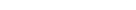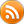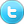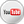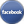# Thread: Help with VFD paramaters to an old 3 phase motor

1. Hello. I've got an issue with my vfd and 3 phase motor. So I've had this before on my 2.2KW vfd.

I'm UK based so 240v 50hz. 3 phase 5/8HP Wadkin motor and a 220V 1HP 1 to 3 phase vfd. I've tried every wiring configuration and you can hear it hum inside the motor and increase as the rpm is increased. I've got 26Ω over each winding and I saw it run before it was disconnected from it's 3 phase supply.

This is the guide for said VFD - https://cdn.hackaday.io//VFD%20ASKP...1%20UserGuide

My parameters are as;
pn1 = 1
pn2 = 400
pn3 = 2
pn4 = 1
pn5 = 3
pn6 = 2
pn7 = 1
pn8 = 8
pn9 = 3
pn10 = 400
pn11 = 100
pn12 = 400
pn13 = 0
pn14 = 80
pn15 = 30
pn16 = 0
pn17 = 30
pn18 = 0
pn19 = 1
pn20 = 10
pn21 = 20
pn22 = 30
pn23 = 40
pn24 = 50
pn25 = 60
pn26 = 70
pn27= 10
pn28 = 3
pn29= 2
pn30 = 2
pn31 = 1
pn32 = 1Reply With Quote

2. Disregard. Issue now resolved.Reply With Quote

####Posting Permissions

• You may not post new threads
• You may not post replies
• You may not post attachments
• You may not edit your posts
•